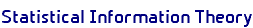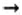Home / Research Interests / Statistical Information TheoryStatistical Information TheoryIn the 1950s and 1960s, there was a considerable effort in information theory to find bounds, for any of various given channels, on the probability of error, Pe , of the best code of block length n and code rate R. For large block lengths, n, this entailed bounds on the function

 E(R) = lim -ln Pe(n, R)/n n(using lim sup and lim inf for upper and lower bounds as appropriate). Under certain simplistic ("very noisy") circumstances, the function E(R) attained the form shown in the figure: linear over a region from 0 to the a critical value called Rcrit, and then roughly parabolic over a region of rates from Rcrit to Shannon's capacity, C, at which point E(R) becomes 0. Many results of this type appear in the complete works of Claude Shannon, including his last papers  on information theory, which were coauthored by Gallager and Berlekamp.

Berlekamp  studied a closely related problem, which was subsequently independently popularized by Stan Ulam and became known as "Ulam's problem", or "20 questions with lies". In this problem one player selects an object at random from a set of M possibilities. His opponent attempts to discover the object by asking n yes-no questions, sequentially. The answerer is permitted to lie up to e times. What values of M, n, and e, are wins for the selector, and which are wins for the questioner? Berlekamp essentially solved the problem for large values of the parameters. Let R = lg M/n, and let f = e/n. Then the boundary values lie on the curve f(R), which is depicted above. Although Berlekamp's paper was often cited in work on "Ulam's problem" in the 1980s and early 1990s, the last half of the paper was never carefully read. Ulam popularized two values of M, namely 1,000,000 and 220. For both of these values of M, for each e, Ray Hill subsequently determined the optimum value of n precisely. Des Jardins then extended these precise results much further. For many values of n and e, he was able to determine the maximum value of M to within one or two objects.

E. R. Berlekamp, "Block Coding for the Binary Symmetry Channel with Noiseless, Delayless Feeback", pages 61-68 in Error-Correcting Codes, edited by H. B. Mann; John Wiley and Sons, New York, 1968; Math. Rev. 38:3072.

E. R. Berlekamp, "The Performance of Block Codes", Notices of the AMS, January 2002, 17-22.

Claude Elwood Shannon, Collected Papers, edited by N. J. A. Sloane and A. Wyner, IEEE Press, New York, 1993, pages 385-454.

David DesJardins, UC Berkeley math PhD thesis, Fall 2001.